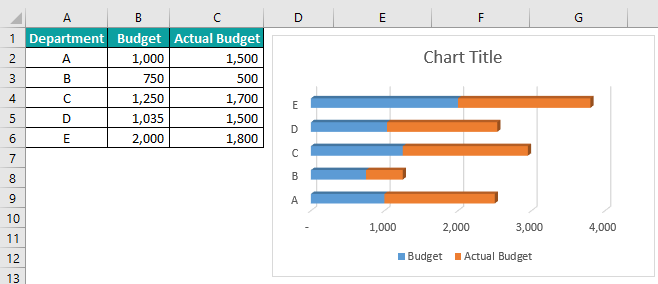# Bar Chart In ExcelArticle byExcelMojo Team## What Is Bar Chart In Excel?

The Bar Chart in Excel is the easiest chart we can create by selecting the parameters and values. Remember, we have to assign one value for each parameter. The Bar Graph can be accessed from the Insert tab under the Charts group. It has different Bar Charts such as clustered bars, stacked bars, and 100% stacked bars in 2D and 3D types. For example, column A shows the value of three items. Therefore, we need to use the below steps to create one.

The steps used to create a bar chart in Excel are as follows:

Go to the Insert tab. Click the drop-down button of the Insert Column or Bar Chart option from the Charts group. Select the Excel Bar Graph type from the drop-down list.

The Chart Design tab is created. Choose the Bar Graph types from the Chart Style group. We will get a Bar Chart as shown in the following image.

###### Key Takeaways
• The Bar Chart type can be changed with the change chart type option in the Chart Design tab.
• The Bar Graph can have titles of the data that will appear in the chart. For example, each data column can use category titles and an overall chart title.
• The information on a chart should be correct; we should ensure our data is laid out correctly.
• Before creating a chart, we must have some data stored in the cells of a worksheet, and we can keep it in any number of worksheets. The worksheets can even be in diﬀerent workbooks.
• ­­­­We can customize the color of each bar of the chart in a different color.

### Steps To Create Bar Chart/Graph In Excel

Step 1: Select the table.

Step 2: Go to the Insert tab.

Step 3: Click the drop-down button of the Insert Column or Bar Chart option from the Charts group.

Step 4: Select the type of chart from the drop-down list.

### Formatting An Excel Bar Chart

• Select the Chart that we want to format.
• Go to the Insert.
• Select the Insert Column or Bar Chart option from Charts.
• The Chart Design tab appears.
• Select the required Bar Chart type from the Chart Styles

### Types Of Bar Chart In Excel

There are two types of graphs such as 2-D Bar and 3-D Bar charts in excel.

#### Example #1 – 2D Bar Chart

For example, the below table shows the price details of fruits. But, first, let us understand the steps to insert Bar Chart in Excel.

In the table,

• Column A lists the fruits
• Column B indicates the cost price
• Column C shows the selling price
• Column D has the calculated profit

The steps to insert Bar Chart in Excel are as follows:

Step 1: Select the data to create a Bar Chart.

Step 2: Go to the Insert tab.

Step 3: Choose the Bar Chart type from the drop-down list under the Charts group.

As soon as we select the style, the Chart Design tab appears.

Step 4: Select the Bar Chart styles from the Chart Styles group. The Bar graph is created as shown in the following image.

Likewise, we can insert a bar chart in Excel.

#### Example #2 – 3D Bar Chart

For example, the following table shows students’ marks in five subjects. But, first, let us understand the steps to create a Bar Graph in Excel.

In the table,

• Column A lists the subjects
• Columns B, C, D, and E show the marks of John, Ron, Harry, and Jenifer, respectively.
• Column F indicates the total marks

The steps to add Bar graph in Excel are as follows:

Step 1: Select the data to create a Bar Chart.

Step 2: Go to the Insert tab.

Step 3: Select the Insert Column or Bar Chart option from the Charts group.

Step 4: Choose the desired bar chart type we want from the drop-down list.

Step 5: The Chart Design tab appears.

Step 6: Select the Bar Chart type from the Chart Style group.

The Bar Chart is formed as shown in the following image:

Similarly, we can create a 3D bar chart in Excel.

#### Example #3

The table below shows employees’ names, annual salaries, and deduction rates in columns A, B, and C. First, let us learn how to show percentages in Bar Chart in Excel.

The steps to insert Percentage in Bar Chart in Excel are as follows:

Step 1: Select the data to create a Bar Chart.

Step 2: Go to the Insert tab.

Step 3: Click the down arrow button of the Insert Column or Bar Chart option from the Charts group.

Step 4: Select the bar chart type from the drop-down list.

Step 5: The Chart Design tab appears.

Step 6: Select the Bar Chart type from the Chart Style group.

We can see Stacked Bar Chart in Excel, as shown in the following image.

Similarly, we can create a percentage in the bar graph in Excel.

#### Example #4

For example, the following table shows the inventory details of fruits. But, first, let us understand how to change the width of the Bar Chart in Excel.

In the table,

• Column A lists the name of fruits
• Column B indicates the amount in stock
• Column C shows the deduction rate

The steps to change the width of the Bar Graph in Excel are as follows:

Step 1: Select the data to create a Bar Chart.

Step 2: Go to the Insert tab.

Step 3: Select the Insert Column or Bar Chart option from the Charts group.

Step 4: Select the formatting of an Excel Bar Chart we want from the drop-down list.

Step 5: The Chart Design tab is formed.

Step 6: Select the format of the Bar Chart from the Chart Style group.

Step 7: Select the bars on the Bar Chart.

Step 8: Click the ‘+’ sign found on the right.

Step 9: Click the right arrow of the Axes option from the list.

Step 10: Click the More Options… option from the list.

Step 11: The Format Axis window opens on the right of the screen.

Step 12: Change the Width of the Bar from the 3-D Format list. The Width of the Bars will be changed, as shown in the following image.

Likewise, we can change the width of the bar chart in Excel.

### Uses Of Excel Bar Chart

An Excel Bar chart forms clustered data with too many categories. But it may become visually complex when categories or series are added. Also, clustered column charts can be difficult to interpret.

The category labels make it easier to read and understand. In addition, it is easy to add data labels at the end of bars. Then, like a column chart, we can include any data series and stack the bars from left to right.

Also, it is useful in showing data changes over time.

### Important Things To Note

• Bar Graph represent small data visually.
• Column charts and Bar Charts may look alike, but the vertical and horizontal axes are interchanged in both charts.
• The data should be arranged very systematically for the correct formation of the chart. If it is not in a suitable format, we cannot create a Bar Chart in Excel.
• Remember, the Bar Chart accepts only numerical values and ignores the non-numerical value, i.e., text or special characters.
• The blank cells between rows or columns are ignored while forming Bar Chart.
• Make the data structure in a simple grid of rows and columns.

What is Bar Chart in Excel?

The Bar Chart in Excel is one of the available chart types. We can create it using parameters and values.

How to make a bar chart in Excel?

Step 1: Select the table to create a bar chart.

Step 2: Go to the Insert tab.

Step 3: Select the Insert Column or Bar Chart option from the Charts group.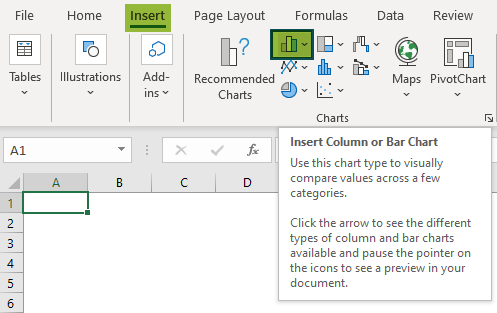Step 4: Select the type of chart from the drop-down list.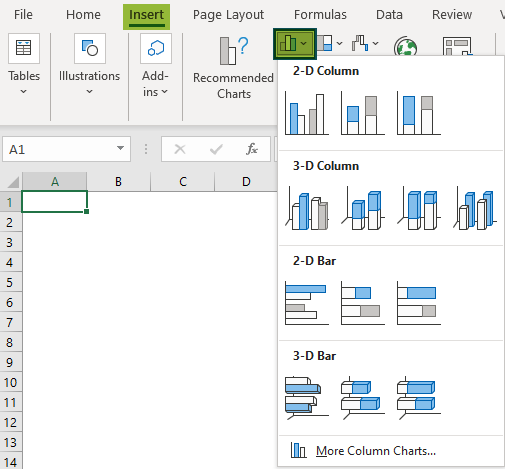How to create Stacked Bar Chart in Excel?

For example, the below table shows a company’s departments, allotted budget, and the actual budget in columns A, B, and C, respectively. But, first, let us learn how to create Stacked Bar Chart in Excel.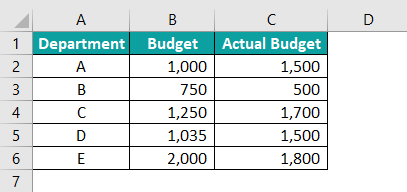The steps to insert Stacked Bar Chart in Excel are as follows:

Step 1: Select the data to create a Bar Chart.

Step 2: Go to the Insert tab.

Step 3: Select the Insert Column or Bar Chart option from the Charts group.

Step 4: Choose the required Excel Bar Chart formatting options from the drop-down list.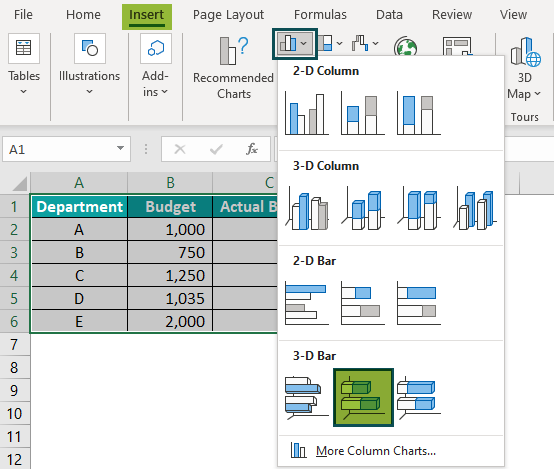Step 5: The Chart Design tab appears.

Step 6: Select the format of the Bar Chart from the Chart Style group.
The Stacked Bar Chart is formed as shown in the following image.